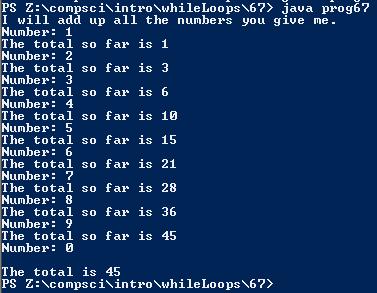# Assignemnt #67, Adding Values in a Loop

## Code

```      /// Name: Alex Pinder
/// Period: 5
/// Program Name: Adding Values in a Loop
/// File Name: prog67.java
/// Date Complete: 1/14/16

import java.util.Scanner;

public class prog67
{
public static void main( String[] args )
{
Scanner keyboard = new Scanner(System.in);

int total, x;

System.out.println( "I will add up all the numbers you give me.");
System.out.print( "Number: " );
x = keyboard.nextInt();
total = x;

System.out.println( "The total so far is " + total );

while ( x != 0 )
{
System.out.print( "Number: " );
x = keyboard.nextInt();
total = total + x;

if ( x != 0 )
System.out.println( "The total so far is " + total );
}

System.out.println( "\nThe total is " + total );
}
}
```

### Picture of the output